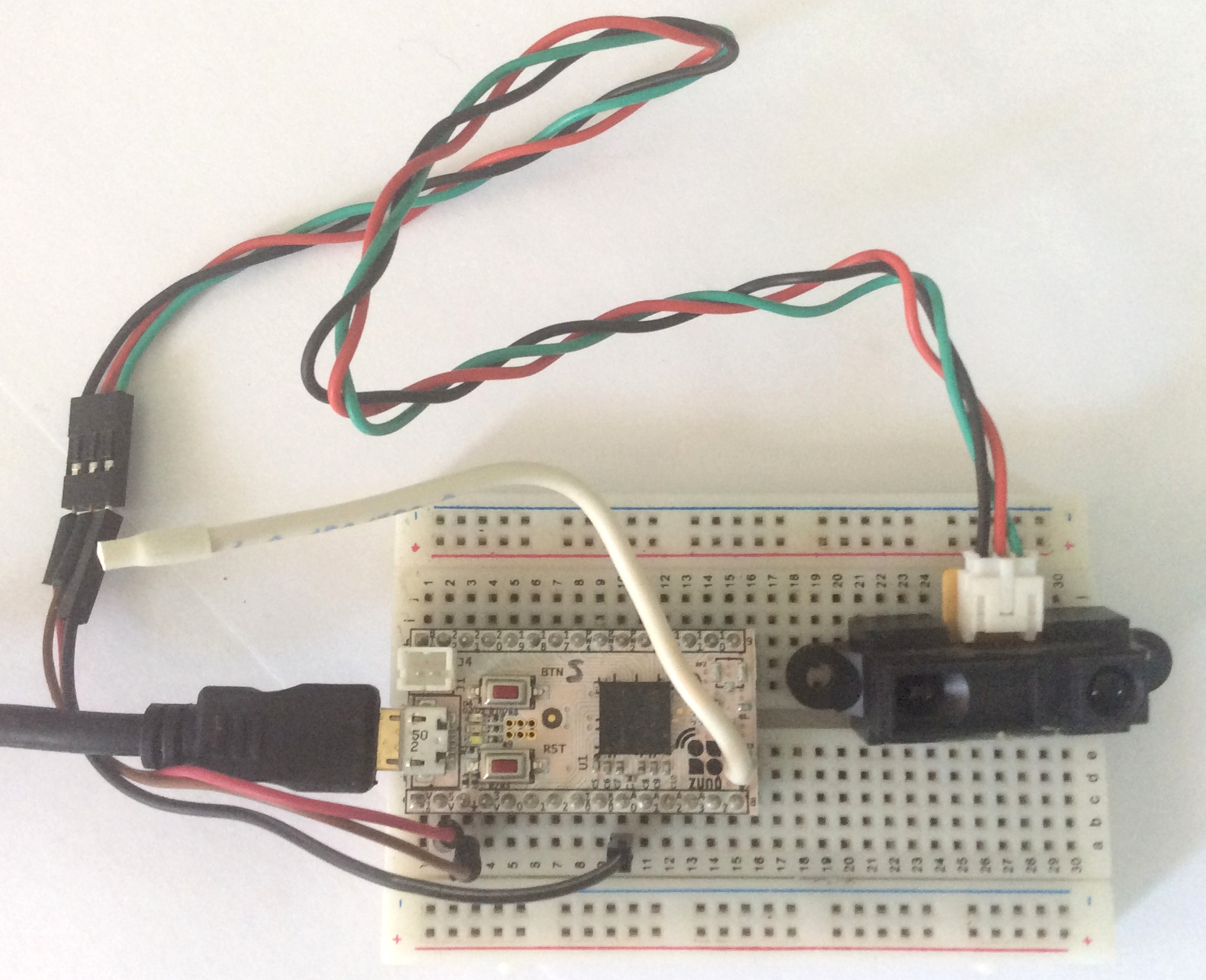# Infrared Proximity Sensor Sharp GP2Y0A21YK

This sketch shows how to connect Infrared Proximity Sensor Sharp GP2Y0A21YK to the Z-Uno board. Distance values are read from sensorand periodically reported to channel Multilevel Sensor.
• Z-Uno board
• Infrared Proximity Sensor Sharp GP2Y0A21YK (like this or this)
• 3 wires``````// demo sketch for connecting Infrared Proximity Sensor Sharp GP2Y0A21YK to Z-Uno

// pin connection to Sharp IR
#define PIN_SHARP A0

// supply voltage (if USB - 3.05 V)
float V = 3.05;

// the number of measurements for smoothing (averaging)
#define COUNT_AVERAGE 10

// set up channel
ZUNO_SETUP_CHANNELS(
ZUNO_SENSOR_MULTILEVEL(ZUNO_SENSOR_MULTILEVEL_TYPE_DISTANCE,
SENSOR_MULTILEVEL_SCALE_METER,
SENSOR_MULTILEVEL_SIZE_TWO_BYTES,
SENSOR_MULTILEVEL_PRECISION_TWO_DECIMALS,
getterDistance)
);

// here we will store the average analog value

// here we will store the value of the distance
float distance;

void setup() {
Serial.begin();
Serial.println("start");
}

void loop() {

// sensor measuring range: 10-80 cm
distance = min(distance, 80);
distance = max(distance, 10);
Serial.print("distance = ");
Serial.println(distance);

// send data to channel
zunoSendReport(1);
// every 30 second
delay(30000);
}

// function analog value transformation to the distance (cm)
int getDistance(int avalue) {
float volts;

volts = avalue * 0.0048828125 / 5 * V;
Serial.print("volts = ");
Serial.println(volts);

return 32 * pow(volts, -1.10);
}

// Obtain readings from the sensor and average to smooth
int averaging = 0;  // variable for adding data
int avalue1;

// We do COUNT AVERAGE measurement
for (int i = 0; i < COUNT_AVERAGE; i++) {